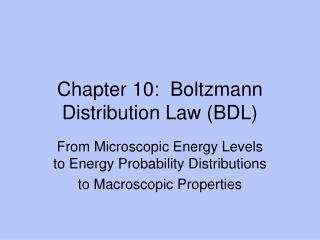# Chapter 10: Boltzmann Distribution Law (BDL) - PowerPoint PPT PresentationDownload PresentationChapter 10: Boltzmann Distribution Law (BDL)

Chapter 10: Boltzmann Distribution Law (BDL)Download Presentation## Chapter 10: Boltzmann Distribution Law (BDL)

- - - - - - - - - - - - - - - - - - - - - - - - - - - E N D - - - - - - - - - - - - - - - - - - - - - - - - - - -
##### Presentation Transcript

1. Chapter 10: Boltzmann Distribution Law (BDL) From Microscopic Energy Levels to Energy Probability Distributions to Macroscopic Properties

2. I. Probability Distribution • Microstate: a specific configuration of atoms (Fig 10.1 has 5 microstates) • Macrostate: a collection of microstates at the same energy (Fig 10.1 has 2 macrostates); they differ in a property determined by finding <Property>. • Recall: <Prop> requires the probability distribution (i.e. weighting term) for the system.

3. II. BDL • Consider a system of N atoms with allowed energy levels E1, E2, E3, …Ej… • These energies are independent of T. • What is the set of equilibrium probabilities for an atom having a particular energy? p1, p2, …pj,…, i.e. what is the probability distribution?

4. BDL • Let T, V, N be constant  dF = 0 is the condition for equilibrium. • dF = dU –TdS – SdT = dU – TdS = 0 • Find dU and dS; plug into dF and minimize • Σpj = 1  αΣdpj = 0 is the constraint

5. BDL • U = <E> = Σ pjEj • dU = d<E> =Σ(pjdEj + Ejdpj) but dEj = 0 since Ej(V, N) and V and N are constant andEj does not depend on T. • dU = d<E> = Σ(Ejdpj) • S = -k Σpjℓn pj  • dS = -k Σ(1 + ℓn pj)dpj

6. BDL • dF = dU – TdS = 0 Eqn 10.6 = Σ[Ej + kT(1 +ℓn pj*) + α] dpj* = 0 • Solve for pj* = exp(- Ej /kT) exp(- α/kT – 1) • Σpj* = 1 = exp(- α/kT – 1) Σ exp(- Ej /kT) • BDL = pj* = [exp(- Ej /kT)]/Σ exp(- Ej /kT) = [exp(- Ej /kT)]/Q Eqn 10.9 (Prob 6) • Denominator = Q = partition function Eqn 10.10

7. III. Applications of BDL • p(z) = pressure of atm α N(z) α exp (-mgz/kT) • p(vx) = Eqn 10.15 = 1-dimensional velocity distribution = √m/(2πkT) exp (-mvx2/2kT) <vx2> = kT/m for 1-di <Ek> = kT/2 for 1-di; kT for 2-di; 3kT/2 for 3-di • p(v) = [m/(2πkT)]3/2 exp (-mv2/2kT) for 3-di = Eqn 10.17

8. IV. Q = Partition Function • Q = Σ exp(- Ej /kT) • Tells us how particles are distributed or partitioned into the accessible states. (Prob 3) • As T increases, higher energy states are populated and Q  number of accessible states. This is also true for energy levels that are very close together and easily populated. (Fig 10.c)

9. Q • An inverse statement can be made: As T decreases, higher energy states are depopulated and with the lowest state being the only one occupied. In this case, Q  1. This is also true for energy levels that are very far together and only the j = 1 level is populated. (Fig 10.5a)

10. Q • The ratio Ej/kT determines if we are in the high T (ratio is low) range or low T (ratio is high) range. (Fig 10.6) • If the ℓthenergy level is W(Eℓ)-fold degenerate, then Q = ΣW(Eℓ)exp(- Eℓ/kT) • Then pℓ* = W(Eℓ)exp(- Eℓ/kT)/Q

11. Q • A system of two independent and distinguishable particles A and B has Q = qA qB; in general Q = qN • A system of N independent and indistinguishable particles has Q = qN/N! • (Prob 5, 8)

12. V. From Partition Function to Thermodynamic Properties • Recall the role of Ψ ( energy, angular momentum, position, … i.e. properties) in QM. To some extent, the role of Q ( U, S, G, H… i.e. thermo. prop.s) is similar. • Table 10.1 Prob 11 • Ensemble: collection of all possible microstates. Canonical (constant T, V,N), Isobaric-isothermal (T,p,N), Grand canonical (T,V,μ), microcanonical (U,V<N)